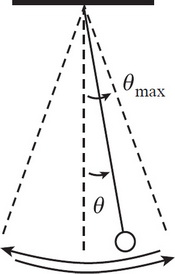# AP Physics 1 Question 28: Answer and Explanation

### Test Information

Question: 28

8.A simple pendulum executes simple harmonic motion as it swings through small angles of oscillation. If θmax denotes the amplitude of the oscillations, which of the following statements is true?

• A. When θ = 0, the tangential acceleration is 0.
• B. When θ = θmax, the tangential acceleration is 0.
• C. When θ = 0, the speed is 0.
• D. When θ = 0, the restoring force is maximized.